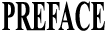What is the Slide Rule ?The Slide Rule is an instrument that may be used for saving time and labour in most of the calculations that occur in practice. By means of the slide rule, one can easily solve with a sufficient degree of accuracy not only all manner of problems involving multiplication and division such as proportion, squares and square roots, cubes and cube roots, but also complicated algebraic and and trigonometrical calculations, without mental strain and in small fractions of the time required to work them out by the usual figuring. For this reason, the slide rule has now become indispensable to students, business men, merchants, engineers, surveyors, draftsmen or estimators.How much education is necessary ?Everyone who has knowledge of decimal fractions can use the slide rule.How long will it take to learn ?Only about half an our will be sufficient for an average person to learn how to use the Hemmi’s Bamboo Slide Rule by carefully reading this short directions. But such knowledge alone will not help him much to realize the merit of the Rule unless accompanied by constant practice, so that the users of the Rule are recommended to repeat practice until well acquainted with it. Short time keenly devoted to the study of its use will enable him to manage the Rule thoroughly, and he will be surprised at its handiness.

Part 1. THE “HEMMI’S” NORMAL SLIDE RULES
 The Scales.The upper scale line of the rule consists of two exactly Similar scales, placed consecutively. The lower scale is like the upper one, but is twice the length.The front of the slide is divided the same as the rule, so that when the 1 of the slide is placed against the 1 of the rule, all the other divisions coincide. The back of the slide is marked with three scales, one being a scale of sines, indicated by the letter S; the second, a scale of tangents, marked T; and the third, a scale of equal parts, for use when the logarithm of a number is required. The Divisions.Each of the upper scales is divided into 10 primary parts, numbered from 1 to 10, which are spaced according to the logarithm of the numbers, and each of these is sub-divided into 10 parts. Between 1 and 2, these last divisions are each divided into 5 parts, and between 2 and 5 into 2 parts.The lower scale, which is used to a much greater

 extent than any other scale on the rule, is like the upper one, first divided into 10 parts, and each of these divided into 10 parts. Between 1 and 2, the sub-division of the main space are each divided into 10 parts; between 2 and 4, they are divided into 5 parts; and for the remainder of the scale, into 2 parts only.The 1 mark on the left hand of the scale may represent 1, 10, 100, 0.1, 0.01, 0.001, etc., the positions of the decimal point being of no importance; but once the value has been—for particular calculation—assigned to it, the ratio of the values must be maintained throughout the scale. Thus, if it is taken to represent 10, the main divisions must be read as 20, 30, 40, etc. The figured sub-divisions of the main space 1-2 will in this case be read as 11, 12, 13, etc., and the small spaced between 1 and 2 will represent 0.1 each, being one-hundredth of the main division, so that the second mark to the right of the 6 figured between 1 and 2 will read as 16.2.Between 2 and 4 the small spaces will represent 0.2 each, being one-fiftieth of the main division and those between.